## 13 September 2017

### Database integration tests are slow? Bullshit!

Probably we all saw projects where running all the tests took about 30 mins, it was possible only using command line, and required remote debugging. That's insane.

But fortunately it's not the only way. Let's do a few tests and see the numbers. By no means it's a benchmark. I just want to show the orders of magnitude. All the following tests use Postgres and run on my old, cheap desktop.

### Sample app: Spring-boot, Flyway, JPA

Let's see a typical spring-boot application with flyway and hibernate jpa:

```@SpringBootApplication
public class Application {
public static void main(String[] args) {SpringApplication.run(Application.class, args);}
}

@Entity @Builder
class SampleEntity {
@GeneratedValue @Id int id;
String name;
int age;
}

@Service
class ExpensiveService {
public ExpensiveService(Repo repo) {
throw new RuntimeException("too expensive for our tests");
}
}

interface Repo extends Repository<SampleEntity, Integer> {
Long countByNameAndAge(String name, int age);
}
```
Of course the startup time of the whole application can be arbitrary long depending on your app.
So as a start let's setup db-only tests.

```@RunWith(SpringRunner.class)
@AutoConfigureTestDatabase(replace = Replace.NONE)
@DataJpaTest
public class RepoTest extends DbTest {

@Autowired Repo repo;
@Autowired TestEntityManager testEntityManager;

@Test
public void should_count_by_age_and_name() {

testEntityManager.persistAndFlush(SampleEntity.builder().age(4).name("john").build());
testEntityManager.persistAndFlush(SampleEntity.builder().age(5).name("john").build());
testEntityManager.persistAndFlush(SampleEntity.builder().age(5).name("john").build());

long count = repo.countByNameAndAge("john", 5);

assertThat(count).isEqualTo(2);
}
}
```

#### Without optimisations

No database is installed, so for sure it will take some time to start one.

After compilation is done, `./gradlew test --rerun-tasks` takes ~14.4s. When we repeat the same test using Spring's `@Repeat(20)`, it takes ~15.2s. Running it from withing IDE using Shift-F10 gives the same results so at least we don't have to switch to console and we can see clickable logs immediately. So what do we see in the logs?

Before the first test starts, spring reports that jvm is running for ~11s. That includes jvm start and building spring context. Building spring context includes, among others, starting and preparing db (~6s), executing flyway's migrations (400ms), setting up hibernate as a jpa provider (~1s)

Each test consists of 3 inserts, a search and a rollback.

first test: ~300ms
next one: ~100ms
every other: ~50ms

#### With running database

The most obvious improvement would be to have db up and running on the development machine. Let's do
```docker run --rm -p 5432:5432 postgres:9.6.1
```
and then again Shift-F10. As expected we just saved 6s. Now, before 1st test, jvm is running ~5s.

#### Without migrations

What else we can improve? Current config clears the database and run flyway migrations before first test. Although 400ms is not much, Flyway's migrations usually grow over time and in bigger projects it can take tens of seconds. Especially because some databases have really slow DDL operations.

Often we work on some new queries and we don't modify db structure. So let's temporary disable db cleanup and therefore the need of flyway's migrations using `.fullClean(__ -> {})` and flyway's verification using environment variable `flyway.enabled=false`. Of course to make it convenient it should be handled by some feature switch but it's just a PoC.

So now, it's ~4,6s and ~50ms for each spring's JPA test. And all running from IDE. Not bad.

### Sample app: No ORM

Much more spectacular results we can get when we don't use any ORM. That's much common in small apps (e.g. microservices). Let's see a simple spring-boot, flyway, jdbc app:
```@SpringBootApplication
@Repository
@AllArgsConstructor(onConstructor = @__(@Autowired))
public class Repo {
final JdbcTemplate jdbcTemplate;

/** fails at first batch containing null */
public void save_ints_in_batches_by_two(List ints) {
jdbcTemplate.batchUpdate("insert into some_table values (?)", ints, 2,
(ps, value) -> ps.setInt(1, value));
}

/** executes stored function */
public int count() {
return jdbcTemplate.queryForObject("select my_count()", Integer.class);
}

public static void main(String[] args) {SpringApplication.run(Repo.class, args);}
}
```
In this case we don't need Spring to run tests:
```public class NgTest {

Repo repo;

@BeforeMethod
public void prepareDb() {
DataSource dataSource = StandaloneDbSetup.prepareDataSourceForTest();
repo = new Repo(new JdbcTemplate(dataSource));
}

@Test
public void should_insert_all_values() {

repo.save_ints_in_batches_by_two(Arrays.asList(1,2,3,4,5));

int count = repo.count();
assertThat(count).isEqualTo(5);
}

@Test
public void should_fail_on_batch_containing_null() {

assertThatThrownBy(() ->
repo.save_ints_in_batches_by_two(Arrays.asList(1,2,3,null,5))
).isNotNull();

int count = repo.count();
assertThat(count).as("only 1st batch of size 2 should have succeeded")
.isEqualTo(2);
}
}
```

On a clean machine it takes ~6.5s. When Postgres is up, running those two tests takes 0.5s.

#### Without Flyway

After completely removing Flyway (migration and validation) using `.buildSchema(__ -> {}).fullClean(__ ->{})`, 2 tests takes: 220ms in total. One of them runs in 7ms. So instead of 2 tests let's run 20: 10 times each by using `@Test(invocationCount = 10)`.

Now, we run 20 tests. There were 2 groups of tests

1. 1 commit + 1 integrity violation; first test took 240ms, others 5-16ms
2. 3 commits; 20-24ms

The whole execution time reported by IDE is less than 1.6s.

And that's all without things like moving database to tmpfs, changing app, etc. All the changes can be done in one test superclass.
Is it slower than normal unit tests? For sure. Is it slow? Not really.

Full source: JPA, standalone.

## 19 April 2016

### Spring's @Value with custom types

Everyone knows you can do:
```@Value("\${some.property}") String myString;
@Value("classpath:/\${some.file}") Resource file;
```
Some know you can also do:
```@Value("classpath:/myFile.txt") InputStream inputStream;
```
But what if we want to do:
```@Value("some string \${with.properties}") MyObject myObject;
```
In this case spring will automatically look for builder methods `valueOf`, `of`, `from` or proper constructor (as described in ObjectToObjectConverter). So
```public MyObject(String parameter) {...}
```
will be enough.

If more complex conversion is needed, one possibility is to create a bean named `conversionService` and type ConversionService with registered converters. For example:
```@Bean(name="conversionService")
public ConversionService conversionService() {
DefaultConversionService conversionService = new DefaultConversionService();
return conversionService;
}
```

Tested with spring 4.2.5.RELEASE

## 10 January 2016

### Remote git branch with different name

Sometimes we want to publish one of our local branches as a master branch in a new git repository.

To initialize newly created github repo add new remote. Let's name it `other-repo`.
```git remote add other-repo https://github.com/xxx/yyy.git
```
To push `local-branch` as remote `master`:
```git push -u other-repo local-branch:master
```
To later push any new commits (this can be simplified using git's config option `push.default`):
```git push other-repo local-branch:master
```
To delete the tracking without deleting remote branch:
```git branch local-branch --unset-upstream
```
To make existing local branch track existing remote branch (master):
```git branch other-repo/master local-branch
```
To see all the trackings:
```git branch -vv
```

git version 1.9.1

## 9 December 2015

### Tips for R beginners

I'm not an R expert but those few things may save you some time. Especially when doing coursera's courses.

#### Installation

Don't install R nor R studio from your system package manager. It's a waste of time. Of course it will work and you'll be able to run hello world but soon you will need some external libraries. And some of them will be outdated others will have conflicting dependencies so the installation will fail. At least that's the case with ubuntu 14.04.

#### rJava problem

Some libraries require java. If you have a problem with 'rJava' library, it's possible that your R installation by default looks for different (older) java version than you actually have installed. in this case you may try:
```sudo R CMD javareconf
```

as described here: http://stackoverflow.com/a/31316527/1100135

#### Changing locale

If, for any reason, you can't change a locale from inside R, you can run whole R with different locale:
```LC_ALL=C rstudio
```

You can read more about it using `man setlocale`. Still, it won't let you use a few different locales at once.

#### Building / transforming formulas

At some point you will want use the power of lazy evaluation and build/transform formulas instead of providing them by hand. Two functions will be usefull: `substitute` and `as.formula`. Let's say we want to build a function that takes all the predictiors (or, more general, some part of formula) and adds the regression variable `y`(other part of formula)

```make.formula <- function(x) as.formula(substitute(t ~ x))
```
and now we can call it using:
```new.formula <- make.formula(x+y*z)
str(new.formula)
```
to get:
```Class 'formula' length 3 t ~ x + y * z
```

#### Tuning knitr rendering

Each code chunk {r } accepts optional parameters that allow you, for example, control if code is executed, if diagnostic messages are also rendered, if computation is cached, if each command prints its output or whole output is displayed at the end etc. Sample:
``````{r cache=T, message=F, results='hold'}
library(randomForest)
system.time(fit <- randomForest(classe ~ ., data=training))
fit
``````
It will exclude diagnostic from loading library, cache trained model and display whole output at the end. Do ?opts_chunk to see the reference page of available options (in `library(knitr)`) and links to the online documentation.

For inline R do: ``r 2 + 3 * x``

#### Benchmarking

```System.time(x <- expensive.function())
```
or to compare multiple computations:
```library(rbenchmark)
benchmark(x <- expensive.function1(), y <-expensive.function2())
```
Above code will do the actual measurement and also will assign new variable in the current environment.

#### Training prediction models with Caret

`train` delegates to other prediction method based on type. Often it's way faster to call directly the underlying method. We may loose all the caret's meta-parameter tuning but still often the model we get is good enough while having the training orders of magnitude faster. Eg:
```train(y ~ x, data=training, method='rf')
randomForest(y ~ x, data=training)
```

## 16 September 2015

### Integer overflow: zero from non-zero multiplication

People sometimes ignore overflow problem because their algorithm is still valid (eg checking if counter has changed within some short period of time). But keep in mind that overflow causes some fundamental math laws don't hold any more. One of them is: when we multiple a few integer numbers we got zero if and only if at least one of the factors is zero. Let's see that in action. Will this loop ever end?
```int x = 1;
while(true) {
x *= RandomUtils.nextInt(1, Integer.MAX_VALUE); // positive random number
if (x == 0) break;
}```
In practice it ends instantly. Even though we multiply only non-zero integers we got a zero as a result. And, of course, it has nothing to do with rounding precision. When we take a closer look, it becomes obvious that to get zero we just have to produce any number that has a binary representation with 32 (in case of java's int) zeros at the end. And `x` accumulates trailing zeros with each multiplication that contains 2 in its prime factors (every second loop on average). So to get zero you can simply multiply two valid ints: (1 << 30) * 4. Same works with any combination of positive and negative numbers: in java's representation negative powers of two also accumulate trailing zeros.

## 5 July 2015

### IoC is not DI

Often I see posts and hear people using interchangeably terms 'inversion of control' and 'dependency injection'. But it's not the same thing.

Inversion of control is a design pattern for, let's say, decoupling 'what' from 'when'. It lets some generic code pass the flow of control to custom components. It increases modularity and extensibility. It's about applying the Hollywood Principle: "Don't call us, we'll call you".

Dependency injection is a design pattern that applies IoC to resolving dependencies. In this pattern a component X doesn't have control over creation of its own dependencies anymore. Instead, the control is inverted and given to another component Y which creates dependencies and inject them into X.

But DI is not the only one realization of IoC. Some others are:

service locator,
aspects,
events / callbacks / event handlers,
strategy,
template method

and those patterns are often used to implement GUI, schedulers, plugins, declarative transactions or many frameworks/libraries (like servlets, junit runners etc).

## 22 April 2015

### Hirschberg's algorithm explanation

Some time ago I needed to implement Hirschberg's algorithm but I couldn't find any good explanation of it. So here is mine attempt.

First of all: It's used to find the optimal sequence alignment. This alignment has to be measured somehow. As a metric we can use, for example, Levenshtein distance. Which means insertion, deletion and change have the same cost. This can be directly translated to computing list of all changes required to change one word into another.

Let's say we have a word L of size n and a word R of size m.

#### Needleman–Wunsch algorithm

First we need to understand Needleman–Wunsch algorithm. It's the easiest way to compute the alignment. The algorithm has just a few lines of code. Its time complexity is O(n*m), space complexity is also O(n*m). This is simple dynamic programming: having edge values we fill the array from top-left to bottom-right corner, choosing minimum (or maximum, depending on the metric. Let's say we are looking for the minimum edit distance). For L = bcd and R = abcde: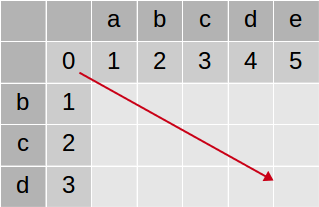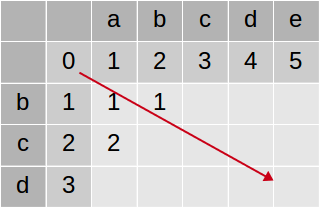After the whole array is filled we go backward (from bottom-right corner) and recreate the path.Each horizontal arrow represents an insertion, vertical - deletion and diagonal - match or replacement (depending if letters match or not). Therefore this array represents alignment:
```- b c d -
| | |
a b c d e
```
It also means that edit distance between bcd and abcde is 2. So we can change one into another with only 2 changes. But it's not all. We can get more information from this table. Last row contains all edit distances (D) between L and all prefixes of R:

D(bcd, abcde) = 2
D(bcd, abcd) = 1
D(bcd, abc) = 2
D(bcd, ab) = 3
D(bcd, a) = 3
D(bcd, ) = 3

If we are interested only in edit distance but not the whole alignment then we can reduce Needleman–Wunsch algorithm space complexity. The key observation is that we can fill the array horizontally (or vertically) so at any time we need only 2 adjacent rows of the array:Its space complexity is O(n) or O(m) (depending on the filling direction) and time complexity remains O(n*m). Let's call this version NW'. If we choose correct direction then last row also contains all edit distances (D) between L and all prefixes of R.

#### Hirschberg's algorithm

Hirschberg's algorithm lets us compute the whole alignment using time O(n*m) and space O(m+n). It uses NW' algorithm and divide and conquer approach:
1. if problem is trivial, compute it:
1. if n or m is 0 then there is n or m insertions or deletions
2. if n = 1 then there is m-1 insertions or deletions and 1 match or change
2. if problem is bigger, divide it into 2 smaller, independent problems
1. divide L in the middle. into 2 sublists L1, L2
2. find optimum division R = R1 R2
3. recursively find the alignment of L1 and R1
4. recursively find the alignment of L2 and R2
5. concatenate results
Of course point 2. needs better explanation. How can we divide big problem into smaller ones? Let's imagine some optimal alignment between L = a1...a27 and R = b1...b32:It means we can transform L into R in 15 operations (to be more precise: cost of transformation is 15). Obviously we can divide this alignment at any arbitrary position into 2 independent alignments, for example: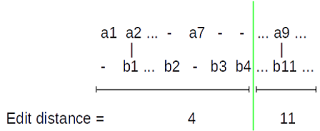Above edit distances is of course just an example. The point is, they must add up to the overall edit distance (15).

So now we have L = (a1...a7) (a8...a27) = L1 L2 and R = (b1...b4) (a8...a32) = R1 R2. It means we can transform L1 into R1 in 4 operations and L2 into R2 in 11 operations. We can concatenate those transformations to get the overall result. It means that if we somehow know the right division we'll be able to compute the alignment of L with R by computing alignments of L1 with R1 and L2 with R2 and simply concatenating results. So it will be possible to split big problem recursively until we reach trivial cases. For example (from wikipedia) to align AGTACGCA and TATGC we could do:
```(AGTACGCA,TATGC)
/              \
(AGTA,TA)            (CGCA,TGC)
/     \              /      \
(AG,)   (TA,TA)      (CG,TG)  (CA,C)
/   \        /   \
(T,T) (A,A)  (C,T) (G,G)
```

But if we have L divided into L1 and L2 how do we know where to divide R? Let's say we want to align (bcdce, abcde)Firstly, let's arbitrary divide L in the middle. So we have two new lists L1 and L2.So where is the correct division of R? Well, we just need to ensure that edit distance (L1, R1) + edit distance (L2, R2) will not be larger than the overall edit distance (L, R). That is, we will use the shortest possible transformation (or alignment). How to ensure that? Well, some of the possible divisions are the optimal ones. So just check all of them and pick the one with the smallest sum of edit distances.
Ok, but how to quickly compute edit distances of L1 with all possible R1 and L2 with all possible R2? Here NW' algorithm can help us. It's straightforward to run for the upper half: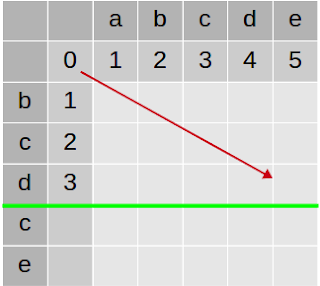After NW' run is completed we have edit distances between L1 and all possible R1:But what about L2 and all possible R2? Again NW' can help, we just need to run it backward. After all edit distance is the same if we look at both words forward or backward, right?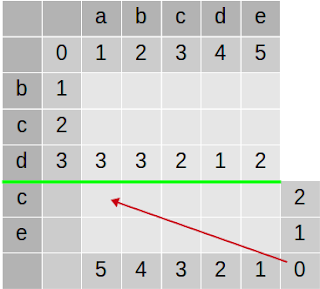After NW' run is completed we have edit distances between L2 and all possible R2:Now, we need to choose the partition of R. Let's say we choose to divide R = abcde into R1 = empty and R2 = abcde:Now we would have to align L1 = bcd with R1 = empty and L2 = ce with R2 = abcde. What would be the edit distances?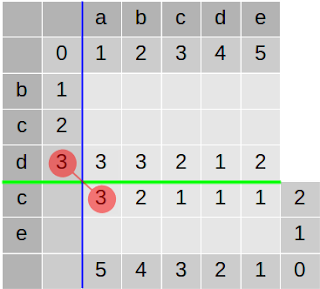It means we can transform L1 (bcd) into R1 (empty) in 3 operations and L2 (ce) into R2 (abcde) in 3 operations. So choosing this division we would transform L into R in 6 operations. In other words cost of alignment of L and R with this division would be 6. Can we do better? What if we place the dividing line elsewhere?Now we would align L1 (bcd) with R1 (a) with cost 3 and L2 (ce) with R2 (bcde) with cost 2. Overall alignment cost (edit distance) would be 3 + 2 = 5. And so on. The last possible position is:Which is aligning L1 (bcd) with R1 (abcde) with cost 2 and L2 (ce) with R2 (empty) with cost 2. Overall cost (distance) is 2+2 = 4. Obviously the smallest overall edit distance is: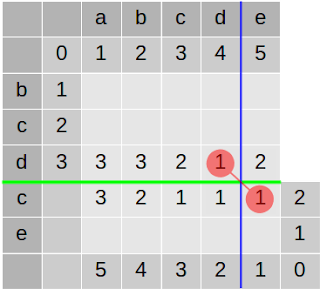So this is the division we were looking for! Now we have 2 independent subproblems: align L1 = bcd with R1 = abcd and L2 = ce with R2 = e. Let's look at the array, it's divided into 4 parts and now we are only interested in top-left and bottom-right part. We will never ever need to look at top-right and bottom-left part. So the overall problem has been reduced by half: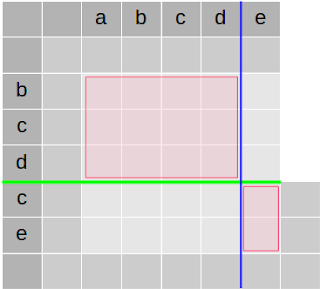That's it. Rest is just basic but a bit tedious math on subarray boundaries and debugging off-by-one errors.

#### Complexity

space: We need to store L and R. Each NW' run uses only 2 rows of size n. Each time we divide L by 2 so the recursion depth is O(log n), Therefore total used space is O(n+m)

time: At each step we run NW' on the whole L x R array. Later 2 subproblems are created of the total size of the half of the original array. So number of operations is: (1 + 1/2 + 1/4 + ...) * (n*m) = O(n*m)